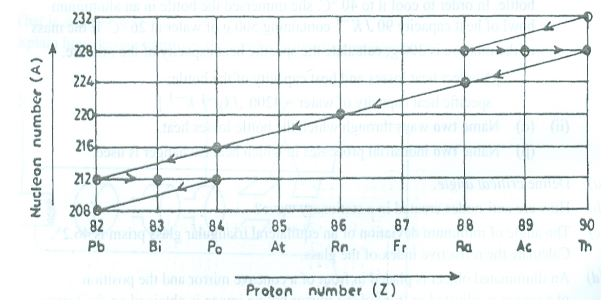Question 12

(a) Explain the following terms:

a. Mass defect;

b. Binding energy of a nucleus.

(b)

(i) Assuming the wave nature of an electron, what is the effect of decreasing the speed of a photoelectron on its; (α) wavelength? (β) energy?

(ii) A particle of mass 4.4 x 10-23 kg moves with a velocity of 105 ms-1. Calculate its wavelength. (h = 6.6 x 10-34 Js)

(c)The diagram above shows part of a radioactive decay series. Use it to answer the following questions.

(i) Name a pair of isotopes

(ii) Name the isotopes with which the series starts.

(iii) Write down a nuclear equation for two examples of each of: (α) alpha decay; (β) beta decay.

Observation

Part (a):    This question was not popular among the candidates. Performance was extremely poor.

Part (b)(i):        The question was poorly attempted and performance was poor.

(ii):        Performance was low among candidates.

Part (c): Performance for (i), (ii), (iii) was generally low. Many candidates were confused about the graph and lack the understanding of the next step to take.